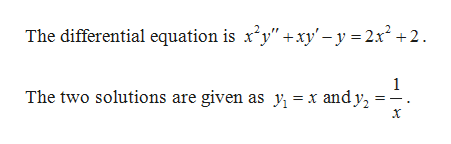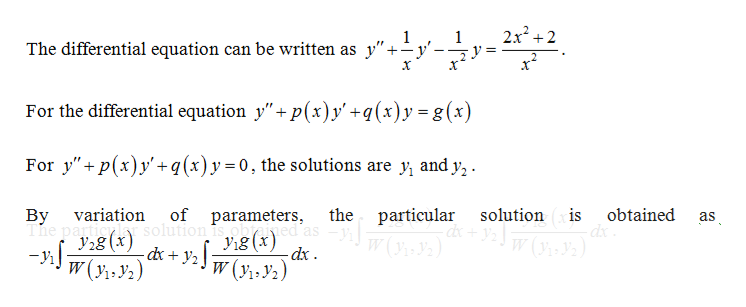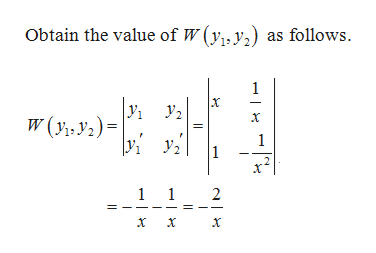Question
check_circle

Step 1

Given:help_outlineImage TranscriptioncloseThe differential equation is x2y" +xy'-y =2x2 +2 1 The two solutions are given as y = x andy2 fullscreen
Step 2

Solution:help_outlineImage Transcriptionclose2x22 1 1 The differential equation can be written as y"+-y' For the differential equation y"+p(x)y'+q(x)y= g(x) For ypxy'+q(x)y= 0, the solutions are y and y, of parameters The particy lar solution is ohted as V1g (x) particular variation the solution is obtained By as y28 (x W(y1 y2) W(Y2) dx fullscreen
Step 3

Calculatio...help_outlineImage TranscriptioncloseObtain the value of W (y, y) as follows 1 У. W(y, y2)= 1 2 1 1 2 х fullscreen

### Want to see the full answer?

See Solution

#### Want to see this answer and more?

Solutions are written by subject experts who are available 24/7. Questions are typically answered within 1 hour.*

See Solution
*Response times may vary by subject and question.
Tagged in

### Math